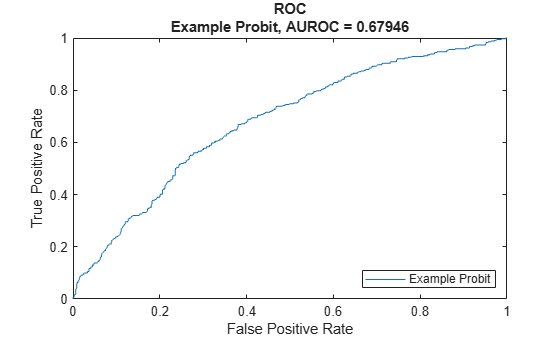# Regression

Create `Regression` model object for loss given default

## Description

Create and analyze a `Regression` model object to calculate the loss given default (LGD) using this workflow:

1. Use `fitLGDModel` to create a `Regression` model object.

2. Use `predict` to predict the LGD.

3. Use `modelDiscrimination` to return AUROC and ROC data. You can plot the results using `modelDiscriminationPlot`.

4. Use `modelAccuracy` to return the R-square, RMSE, correlation, and sample mean error of the predicted and observed LGD data. You can plot the results using `modelAccuracyPlot`.

## Creation

### Syntax

``RegressionLGDModel = fitLGDModel(data,ModelType)``
``RegressionLGDModel = fitLGDModel(___,Name,Value)``

### Description

example

````RegressionLGDModel = fitLGDModel(data,ModelType)` creates a `Regression` LGD model object.```

example

````RegressionLGDModel = fitLGDModel(___,Name,Value)` specifies options using one or more name-value pair arguments in addition to the input arguments in the previous syntax. The optional name-value pair arguments set model object properties. For example, ```lgdModel = fitLGDModel(data,'regression','PredictorVars',{'LTV' 'Age' 'Type'},'ResponseVar','LGD','ResponseTransform','probit','BoundaryTolerance',1e-6)``` creates a `lgdModel` object using a `Regression` model type. ```

### Input Arguments

expand all

Data for loss given default, specified as a table where the first column and all other columns except the last column are `PredictorVars`, the last column is `ResponseVar`.

Data Types: `table`

Model type, specified as a string with the value of `"Regression"` or a character vector with the value of `'Regression'`.

Data Types: `char` | `string`

Name-Value Arguments

Specify optional pairs of arguments as `Name1=Value1,...,NameN=ValueN`, where `Name` is the argument name and `Value` is the corresponding value. Name-value arguments must appear after other arguments, but the order of the pairs does not matter.

Before R2021a, use commas to separate each name and value, and enclose `Name` in quotes.

Example: ```lgdModel = fitLGDModel(data,'regression','PredictorVars',{'LTV' 'Age' 'Type'},'ResponseVar','LGD','ResponseTransform','probit','BoundaryTolerance',1e-6)```

User-defined model ID, specified as the comma-separated pair consisting of `'ModelID'` and a string or character vector. The software uses the `ModelID` text to format outputs and is expected to be short.

Data Types: `string` | `char`

User-defined description for model, specified as the comma-separated pair consisting of `'Description'` and a string or character vector.

Data Types: `string` | `char`

Predictor variables, specified as the comma-separated pair consisting of `'PredictorVars'` and a string array or cell array of character vectors. `PredictorVars` indicates which columns in the `data` input contain the predictor information. By default, `PredictorVars` is set to all the columns in the `data` input except for the `ResponseVar`.

Data Types: `string` | `cell`

Response variable, specified as the comma-separated pair consisting of `'ResponseVar'` and a string or character vector. The response variable contains the LGD data and must be a numeric variable. An LGD value of `0` indicates no loss (full recovery), `1` indicates total loss (no recovery), and values between `0` and `1` indicate a partial loss. By default, the `ResponseVar` is set to the last column of `data`.

Data Types: `string` | `char`

Boundary tolerance, specified as the comma-separated pair consisting of `'BoundaryTolerance'` and a positive scalar numeric. The `BoundaryTolerance` value perturbs the LGD response values away from 0 and 1, before applying a `ResponseTransform`.

Data Types: `double`

Response transform, specified as the comma-separated pair consisting of `'ResponseTransform'` and a character vector or string.

Data Types: `string` | `char`

## Properties

expand all

User-defined model ID, returned as a string.

Data Types: `string`

User-defined description, returned as a string.

Data Types: `string`

Underlying statistical model, returned as a compact linear model object. The compact version of the underlying regression model is an instance of the `classreg.regr.CompactLinearModel` class. For more information, see `fitlm` and `CompactLinearModel`.

Data Types: `CompactLinearModel`

Predictor variables, returned as a string array.

Data Types: `string`

Response variable, returned as a scalar string.

Data Types: `string`

Boundary tolerance, returned as a scalar numeric.

Data Types: `double`

Response transform, returned as a string.

Data Types: `string`

## Object Functions

 `predict` Predict loss given default `modelDiscrimination` Compute AUROC and ROC data `modelDiscriminationPlot` Plot ROC curve `modelAccuracy` Compute R-square, RMSE, correlation, and sample mean error of predicted and observed LGDs `modelAccuracyPlot` Scatter plot of predicted and observed LGDs

## Examples

collapse all

This example shows how to use `fitLGDModel` to create a `Regression` model for loss given default (LGD).

```load LGDData.mat head(data)```
```ans=8×4 table LTV Age Type LGD _______ _______ ___________ _________ 0.89101 0.39716 residential 0.032659 0.70176 2.0939 residential 0.43564 0.72078 2.7948 residential 0.0064766 0.37013 1.237 residential 0.007947 0.36492 2.5818 residential 0 0.796 1.5957 residential 0.14572 0.60203 1.1599 residential 0.025688 0.92005 0.50253 investment 0.063182 ```

Create `Regression` LGD Model

Use `fitLGDModel` to create a `Regression` model using the `data`.

```lgdModel = fitLGDModel(data,'regression',... 'ModelID','Example Probit',... 'Description','Example LGD probit regression model.',... 'PredictorVars',{'LTV' 'Age' 'Type'},... 'ResponseVar','LGD','ResponseTransform','probit','BoundaryTolerance',1e-6); disp(lgdModel)```
``` Regression with properties: ResponseTransform: "probit" BoundaryTolerance: 1.0000e-06 ModelID: "Example Probit" Description: "Example LGD probit regression model." UnderlyingModel: [1x1 classreg.regr.CompactLinearModel] PredictorVars: ["LTV" "Age" "Type"] ResponseVar: "LGD" ```

Display the underlying model. The underlying model's response variable is the probit transformation of the LGD response data. Use the `'ResponseTransform'` and `'BoundaryTolerance'` arguments to modify the transformation.

`disp(lgdModel.UnderlyingModel)`
```Compact linear regression model: LGD_probit ~ 1 + LTV + Age + Type Estimated Coefficients: Estimate SE tStat pValue ________ ________ _______ __________ (Intercept) -2.4011 0.11638 -20.632 2.5277e-89 LTV 1.3777 0.1357 10.153 6.9099e-24 Age -0.58387 0.028183 -20.717 5.2434e-90 Type_investment 0.60006 0.079658 7.5329 6.2863e-14 Number of observations: 3487, Error degrees of freedom: 3483 Root Mean Squared Error: 1.77 R-squared: 0.186, Adjusted R-Squared: 0.186 F-statistic vs. constant model: 266, p-value = 1.87e-155 ```

Predict LGD

For LGD prediction, use `predict`. The LGD model applies the inverse transformation so the predictions are in the LGD scale, not in the transformed scale used to fit the underlying model.

`predictedLGD = predict(lgdModel,data(1:10,:))`
```predictedLGD = 10×1 0.0799 0.0039 0.0012 0.0045 0.0003 0.0127 0.0123 0.2041 0.0200 0.0016 ```

Validate LGD Model

Use `modelDiscriminationPlot` to plot the ROC curve.

`modelDiscriminationPlot(lgdModel,data)`Use `modelAccuracyPlot` to show a scatter plot of the predictions.

`modelAccuracyPlot(lgdModel,data)`